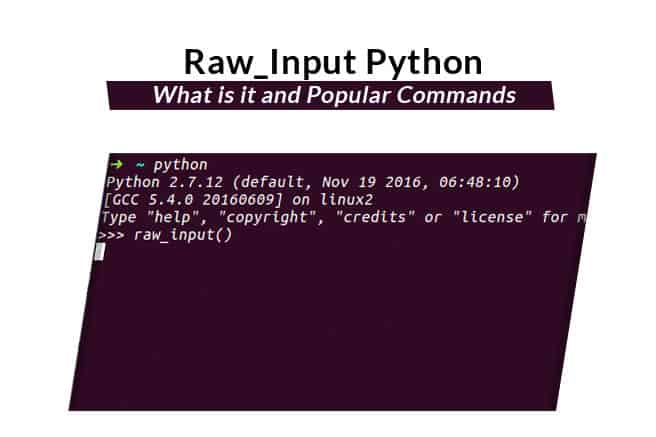×Python is an easy and one the most widely used programming languages around the world.

It is a powerful, general-purpose, object-oriented and high-level programming language. Python 2 is no longer in development and all new features will be added in Python 3.

The Python raw_input is a function that can be used to read data from standard input such as keyboard, converts into a string and returns it.

This will allow us to include user inserted data into a program.

In this tutorial, we will explain how to use python raw_input function on Python version 2 and 3 on Linux.

## Getting Started

Before starting, make sure Python versions 2.x and 3.x are installed on your system.
You can check Python 2 version with the following command:

`python2`
You should get the following output:
` Python 2.7.6 (default, Nov 13 2018, 12:45:42) [GCC 4.8.4] on linux2 Type "help", "copyright", "credits" or "license" for more information. `

To check the Python 3 version, run the following command:
`python3`
You should get the following output:
` Python 3.4.3 (default, Nov 12 2018, 22:25:49) [GCC 4.8.4] on linux Type "help", "copyright", "credits" or "license" for more information. `

## Basic raw_input() Syntax

The basic raw_input syntax for Python version 2.x is shown below:

` mydata = raw_input('Prompt :') print (mydata) `

The basic raw_input syntax for Python version 3.x is shown below:

` mydata = input('Prompt :') print (mydata) `

You can always tell your user what to input by printing a prompt.

## Reading Input with raw_input() for Python 2

There are two versions of input functions used in Python. The input() is used to read data from a standard input in Python 3 and raw_input() is used to read data from a standard input in Python 2.

The raw_input() function treats the received data as string even without quotes ” or “”.

In this section, we will see how to use raw_input() in Python 2. Let’s use raw_input() with some example.

For example, create a Python file that asks a user to input their name, use the raw_input() function to store input value to a variable name then print the value of variable name to standard output.

`nano sample.py`

`#!/usr/bin/python # Version 2 print ("What is your name?") name = raw_input() print ("Hello %s! how are you" % name)`

Save and close the file when you are finished. Then, run the Python script with the following command:

`python sample.py`

You will be asked to provide your name, provide your name and hit Enter. You should see the following screen:## Reading Input with input() for Python 3

In Python version 3.x, you will need to use input() instead of raw_input(). The input() function treats the received data as a string if it is included in quotes ” or “”, otherwise the data is treated as a number.

For example, you will need to modify the sample.py file for Python version 3.x as shown below:

`nano sample.py`

Make the following changes:

`#!/usr/bin/python # Version 3 print ("What is your name?") name = input() print ("Hello %s! how are you" % name)`

Now, run the sample.py file:

`python3 sample.py`

You should see the following screen:## Convert String Value To Integer Value with raw_input() for Python 2

If you want to convert string value to integer value with raw_input() then you will need to use int() function.

Let’s create a test.py file that asked you to provide your mobile number, use the raw_input() function to store input value to a variable name number then print the value of variable name to standard output.

`nano test.py`

`#!/usr/bin/python # Version 2 number = int(raw_input("What is your mobile number ? :")) print(number)`

Save and close the file when you are finished. Then, run the script with the following command:

`python test.py`

You will be asked to provide your mobile number, provide your mobile number and hit Enter. You should see the following screen:## Convert String Value To Integer Value with raw_input() for Python 3

In Python version 3.x, you will need to modify the test.py file for Python version 3.x as shown below:

`nano test.py`

`#!/usr/bin/python # Version 3 number = int(input("What is your mobile number ? :")) print(number)`

Save and close the file when you are finished. Then, run the script with the following command:

`python3 test.py`

You should see the following screen:## Conclusion

In the above tutorial, we learned how to obtain user input from the command line with the raw_input function in Python.

I hope you have now enough knowledge to user raw_input function in Python version 2.x and 3.x.